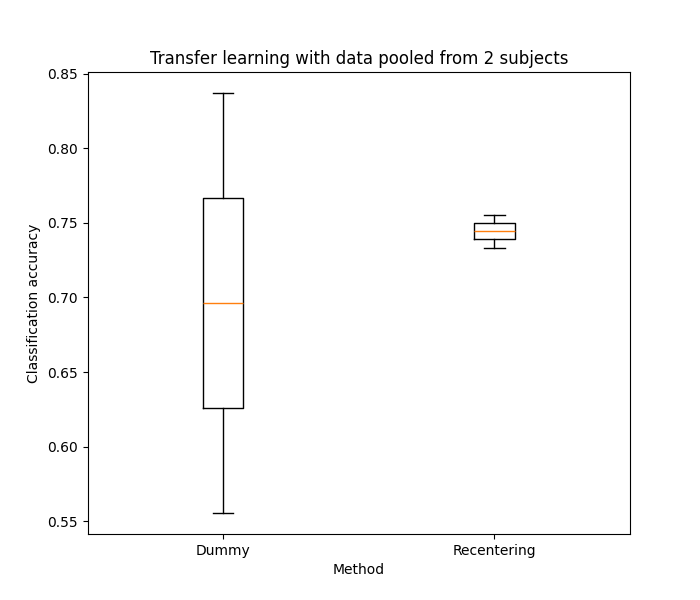# Motor imagery classification by transfer learning¶

In this example, we use transfer learning (TL) to classify epochs from a subject using a classifier trained on data from another subject. We consider TL with a pooling strategy: for each target subject of choice, we use the data from several source subjects to train a single classifier using all of their data points pooled together. We compare the results of simply mixing all covariances from all source subjects without any care (dummy) versus transforming the covariances of all subjects so that they are centered around the Identity matrix (recenter) . We use data from the Physionet BCI database and compare the classification performance of MDM with each strategy.

```import numpy as np
from tqdm import tqdm
from sklearn.pipeline import make_pipeline
from sklearn.model_selection import StratifiedShuffleSplit
import matplotlib.pyplot as plt

from mne import Epochs, pick_types, events_from_annotations
from mne.io import concatenate_raws
from mne.datasets import eegbci
from mne import set_log_level

from pyriemann.classification import MDM
from pyriemann.estimation import Covariances
from pyriemann.transfer import (
encode_domains,
TLDummy,
TLCenter,
TLClassifier,
TLSplitter,
)

set_log_level(verbose=False)
```
```def get_subject_dataset(subject):

# Consider epochs that start 1s after cue onset.
tmin, tmax = 1., 2.
event_id = dict(hands=2, feet=3)
runs = [6, 10, 14]  # motor imagery: hands vs feet

raw_files = [
]
raw = concatenate_raws(raw_files)

# Select only EEG channels
picks = pick_types(
raw.info, meg=False, eeg=True, stim=False, eog=False, exclude='bads')
# select only nine electrodes: F3, Fz, F4, C3, Cz, C4, P3, Pz, P4
picks = picks[[31, 33, 35, 8, 10, 12, 48, 50, 52]]

# Apply band-pass filter
raw.filter(7., 35., method='iir', picks=picks)

# Check the events
events, _ = events_from_annotations(raw, event_id=dict(T1=2, T2=3))

# Define the epochs
epochs = Epochs(
raw,
events,
event_id,
tmin,
tmax,
proj=True,
picks=picks,
baseline=None,
verbose=False)

# Extract the labels for each event
labels = epochs.events[:, -1] - 2

# Compute covariance matrices on scaled data
covs = Covariances().fit_transform(1e6 * epochs.get_data())

return covs, labels
```

We will consider subjects from the Physionet EEG database for which the intra-subject classification has been checked to be > 0.70

```subject_list = [1, 2, 4, 7, 8, 15, 20, 29, 34, 35]

# Load the data from subjects
X, y, d = [], [], []
for i, subject_source in enumerate(subject_list):
X_source_i, y_source_i = get_subject_dataset(subject=subject_source)
X.append(X_source_i)
y.append(y_source_i)
d = d + [f'subject_{subject_source:02}'] * len(X_source_i)
X = np.concatenate(X)
y = np.concatenate(y)
domains = np.array(d)

# Encode the data for transfer learning purposes
X_enc, y_enc = encode_domains(X, y, domains)

# Object for splitting the datasets into training and validation partitions
n_splits = 5  # How many times to split the target domain into train/test
tl_cv = TLSplitter(
target_domain='',
cv=StratifiedShuffleSplit(
n_splits=n_splits, train_size=0.10, random_state=42))

# We consider two types of pipelines for transfer learning
# dct : no transformation of dataset between the domains
# rct : re-center the data points from each domain to the Identity
scores = {meth: [] for meth in ['dummy', 'rct']}

# Base classifier to be wrapped for transfer learning
clf_base = MDM()

# Consider different subjects as target
for subject_target_idx in tqdm(range(len(subject_list))):

# Change the target domain
tl_cv.target_domain = f'subject_{subject_list[subject_target_idx]:02}'

# Create dict for storing results of this particular CV split
scores_cv = {meth: [] for meth in scores.keys()}

# Carry out the cross-validation
for train_idx, test_idx in tl_cv.split(X_enc, y_enc):

# Split the dataset into training and testing
X_enc_train, X_enc_test = X_enc[train_idx], X_enc[test_idx]
y_enc_train, y_enc_test = y_enc[train_idx], y_enc[test_idx]

# (1) Dummy pipeline: no transfer learning at all.
# Classifier is trained only with points from the source domain.
domain_weight_dummy = {}
for d in np.unique(domains):
domain_weight_dummy[d] = 1.0
domain_weight_dummy[tl_cv.target_domain] = 0.0

pipeline = make_pipeline(
TLDummy(),
TLClassifier(
target_domain=tl_cv.target_domain,
estimator=clf_base,
domain_weight=domain_weight_dummy,
),
)

# Fit and get accuracy score
pipeline.fit(X_enc_train, y_enc_train)
scores_cv['dummy'].append(pipeline.score(X_enc_test, y_enc_test))

# (2) Recentering pipeline: recenter the data from each domain to
# identity _.
# Classifier is trained only with points from the source domain.
domain_weight_rct = {}
for d in np.unique(domains):
domain_weight_rct[d] = 1.0
domain_weight_rct[tl_cv.target_domain] = 0.0

pipeline = make_pipeline(
TLCenter(target_domain=tl_cv.target_domain),
TLClassifier(
target_domain=tl_cv.target_domain,
estimator=clf_base,
domain_weight=domain_weight_rct,
),
)

pipeline.fit(X_enc_train, y_enc_train)
scores_cv['rct'].append(pipeline.score(X_enc_test, y_enc_test))

for meth in scores.keys():
scores[meth].append(np.mean(scores_cv[meth]))
```
```  0%|          | 0/10 [00:00<?, ?it/s]
10%|█         | 1/10 [00:02<00:23,  2.60s/it]
20%|██        | 2/10 [00:05<00:20,  2.59s/it]
30%|███       | 3/10 [00:07<00:18,  2.62s/it]
40%|████      | 4/10 [00:10<00:15,  2.51s/it]
50%|█████     | 5/10 [00:12<00:12,  2.49s/it]
60%|██████    | 6/10 [00:15<00:10,  2.53s/it]
70%|███████   | 7/10 [00:17<00:07,  2.54s/it]
80%|████████  | 8/10 [00:20<00:05,  2.62s/it]
90%|█████████ | 9/10 [00:23<00:02,  2.61s/it]
100%|██████████| 10/10 [00:25<00:00,  2.58s/it]
100%|██████████| 10/10 [00:25<00:00,  2.57s/it]
```

Plot results

```fig, ax = plt.subplots(figsize=(7, 6))
ax.boxplot(x=[scores[meth] for meth in scores.keys()])
ax.set_ylim(0.45, 1.00)
ax.set_xticklabels(['Dummy', 'Recentering'])
ax.set_ylabel('Classification accuracy')
ax.set_xlabel('Method')
ax.set_title('Transfer learning with data pooled from 10 subjects')

plt.show()
```